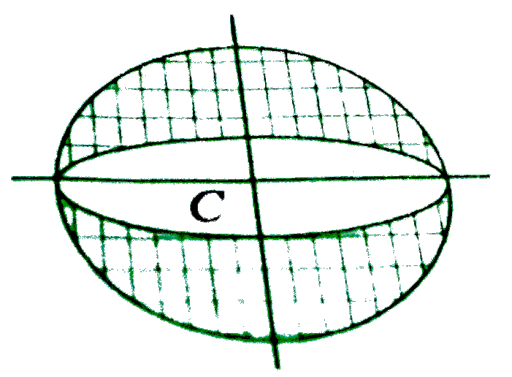There are some experiment in which the outcomes cannot be identifi | FiloClass 12

Math

Algebra

Probability I538

There are some experiment in which the outcomes cannot be identified discretely. For example, an ellipse of eccentricity is inscribed in a circle and a point within the circle is chosen at random. Now, we want to find the probability that this point lies outside the ellipse. Then, the point must lie in the shaded region shown in Figure. Let the radius of the circle be a and length of minor axis of the ellipse be 2b. Given that

Then, the area of circle serves as sample space and area of the shaded region represents the area for favorable cases. Then, required probability isTwo persons A and B agree to meet at a place between 5 and 6 pm. The first one to arrive waits for 20 min and then leave. If the time of their arrival be independant and at random, then the probability that A and B meet is538Connecting you to a tutor in 60 seconds.